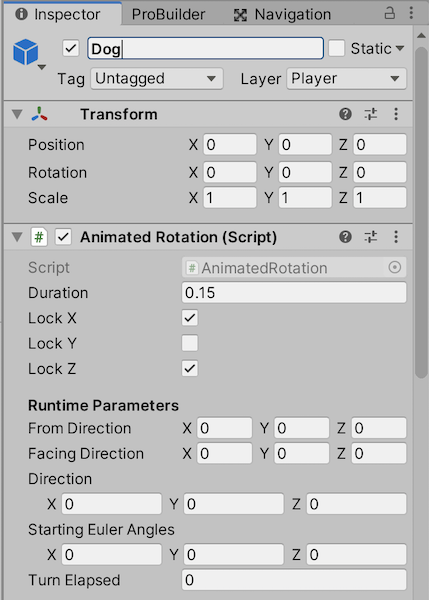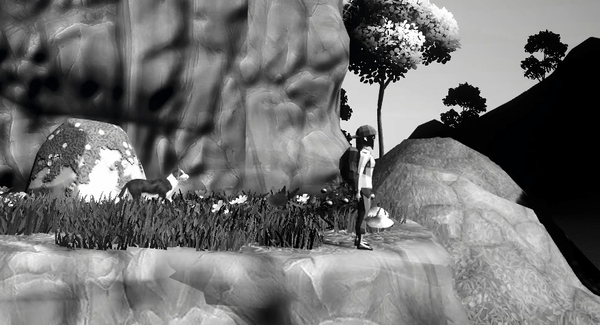# Animating Rotations through Code in Unity

Written by @kylewbanks on Aug 19, 2020.

Unity comes with a variety of ways to spin and rotate GameObjects. If you want to spin or turn to look in a predefined direction then you can use an AnimationClip. If you want your object to look at a particular world position or another object of interest then you have Transform.LookAt. The Quaternion class also provides a dozen functions to work with rotations. There are more ways to rotate an object in Unity than I care to list here, but my point is you have many options.

The problem is, the AnimationClip approach means you need to predefine all the possible rotations, and the LookAt approach isn’t animated so your GameObject is going to immediately snap to the specifed rotation. Same goes for the Quaternion functions.

What if you want to have an object turn to look in a direction (left, right, down, up, etc.) or look at a specific world point, such as another object of interest, and you want to accomplish this dynamically at runtime and with animations so that your objects don’t snap to a new rotation but rather rotate over time?

I’ll show you how I’ve implemented a general AnimatedRotation script which allows you to accomplish these two requirements, and also allows you to lock rotation along particular axes, at runtime. The solution is pretty straightforward and quite reusable, so I hope you’ll get a lot of use out of it.

Let’s jump into it!

## The AnimatedRotation Script

Let’s create a new AnimatedRotation script and assign it to the GameObject you want to rotate. The full script is available at the bottom of the post, but here we’ll go section by section to understand how it works.

First let’s define some variables, including a public Duration field which is the time (in seconds) it takes to turn. I set this to something small for my characters, 0.15 in the GIFs down below, but it will depend on your usecase and how quickly you want to rotate. We have three more public variables, each bools, which will allow us to lock rotations on certain axes so that we don’t get strange results, such as humanoid characters doing sommersaults. Again, your use of these will depend on what you’re trying to rotate and on which axes you want to allow rotation.

```using UnityEngine;

public class AnimatedRotation : MonoBehaviour
{

public float Duration;

public bool LockX;
public bool LockY;
public bool LockZ;

[SerializeField] private Quaternion _fromDirection;
[SerializeField] private Quaternion _targetDirection;
[SerializeField] private float _timeRemaining;
[SerializeField] private Vector3 _startingEulerAngles;

}
```

Next there are some private fields to keep track of the state of the rotation, including the start and end rotations which are named _fromDirection and _targetDirection respectively, and a _timeRemaining float to keep track of how much longer we’ll be rotating. We also have a variable to store the starting euler angles of the object so that we can implement the axes locking, which I’ve named _startingEulerAngles.

If you’re curious about the use of Header and SerializeField attributes on the private fields, check out this post on accessing private and protected fields from the Inspector in Unity. It’s a handy trick for debugging private variables, but you don’t have to use it.

In the Start function we’ll capture the euler angles of the object using transform.localEulerAngles, which will allow us to implement the axes locking when we rotate the object.

```void Start()
{
this._startingEulerAngles = this.transform.localEulerAngles;
}
```

Next we have the Update function:

```void Update()
{
if (this._timeRemaining <= 0f)
{
return;
}
this._timeRemaining -= Time.deltaTime;

// Interpolate towards the target direction
this.transform.rotation = Quaternion.Lerp(
this._fromDirection,
this._targetDirection,
(this.Duration - this._timeRemaining) / this.Duration
);

// Lock axes as needed
Vector3 eulerAngles = this.transform.localEulerAngles;
if (LockX)
{
eulerAngles.x = this._startingEulerAngles.x;
}
if (LockY)
{
eulerAngles.y = this._startingEulerAngles.y;
}
if (LockZ)
{
eulerAngles.z = this._startingEulerAngles.z;
}
this.transform.localEulerAngles = eulerAngles;
}
```

We first check if there’s any time remaining in the rotation and if not, simply return as there’s nothing to do. Assuming there is time remaining, we update the _timeRemaining by subtracting the Time.deltaTime from it.

After the _timeRemaining management we have the main logic where we update the transform.rotation using a Quaternion.Lerp function which linearly interpolates between two Quaternion values.

As an aside before we get into the main rotation logic, if you’re not familiar with interpolators then all that you need to understand is that at their simplest they return a value somewhere between a and b based on the value of a t parameter which is typically a percentage or ratio. For instance, a linear interpolator when given a = 0 and b = 5 will return the following values based on t:

``````0 when t = 0.0
1.25 when t = 0.25
2.5 when t = 0.5
3.75 when t = 0.75
5 when t = 1.0
``````

You can better understand the implementation of a linear interpolator (or lerp) with the following function:

```// EXAMPLE ONLY
// you don't need this for the AnimatedRotation script

float Lerp(float a, float b, float t)
{
return a + ((b - a) * t)
}
```

Unity comes with a variety of interpolators built-in to interpolate between floats, vectors, quaternions, etc. You may have seen functions like Mathf.Lerp, Vector3.Lerp, or Mathf.SmoothStep which is an interpolator with smoothing at the beginning and end so you don’t have a fully linear transition.

For the call to Quaternion.Lerp we use the _fromDirection quaternion as the a parameter, and the _targetDirection as the b parameter. For t we use the amount of time that’s elapsed as a percentage of the total duration, so that as time passed we move further away from a and towards b. At the first iteration we’ll remain at a rotation and by the end we’ll arrive at the b rotation, or _fromDirection and _targetDirection as we’ve named them.

After rotating we check if we want to lock any of the axes, and if so we force the euler angles back to the starting value so that we don’t get rotation on that particular axis. This is particularly useful for upright standing objects or characters where you don’t want them to ever rotate on their side.

Finally, let’s add a public SetDirection function which allows us to specify what direction we want to turn towards:

```public void SetDirection(Vector3 direction)
{
this._timeRemaining = this.Duration;
this._fromDirection = this.transform.rotation;
this._targetDirection = Quaternion.FromToRotation(
Vector3.forward,
direction
);
}
```

Here we reset the _timeRemaining to be the full Duration and capture the current rotation as the _fromDirection so we can keep that as our a value for the Quaternion.Lerp call. We set _targetDirection equal to the result of Quaternion.FromToRotation which basically says we want a rotation that points the forward direction (or z-axis) towards the specified direction. Just as an example to help understand this function, if you wanted the object to face the direction using it’s y-axis you could use Vector3.up instead of Vector3.forward. Typically though we want the object to look in a direction such that it’s z-axis or forward direction is facing the target.

With the script setup, go ahead and add it as a component to your GameObject and don’t forget to supply a value for the Duration parameter and lock the appropriate axes. For this dog object I only want to rotate on the Y-axis so I’ll lock the others, and give it a duration of 0.15.Note: the full script is available at the bottom of the page if you had any trouble following along.

## Setting the Direction

There are two ways to use this script, the first of which is by using global directions via the Vector3 constants such as:

• Vector3.right
• Vector3.left
• Vector3.up
• Vector3.down
• etc.

This will result in your object turning to look in these global directions as opposed to looking at a more specific location. For example the following script will turn left or right based on user input using the arrow or A and D keys:

```AnimatedRotation animatedRotation = this.GetComponent<AnimatedRotation>();

float xInput = Input.GetAxisRaw("Horizontal");
if (xInput > float.Epsilon)
{
animatedRotation.SetDirection(Vector3.right);
}
else if (xInput < -float.Epsilon)
{
animatedRotation.SetDirection(Vector3.left);
}
```

With this you get a result like the following, where the dog quickly spins around whenever the input changes directions:But what if you want to look at a specific location, such as a GameObject or Transform somewhere in the scene. You can do this by using the difference between the position of the target object and the position of the object to rotate. For instance, the following example would turn to look at the player:

```AnimatedRotation animatedRotation = this.GetComponent<AnimatedRotation>();

GameObject objectToLookAt = GameObject.FindGameObjectWithTag("Player");

animatedRotation.SetDirection(
objectToLookAt.transform.position - animatedRotation.gameObject.transform.position
);
```

Now the AnimatedRotation will look at the position of the objectToLookAt. This is actually how I’m handling many of the rotations in my Untitled game, including for some of the NPC interactions, as you can see here when the dog walks up beside the girl and she turns to look at him:## Full Script

Here’s the full script ready to go. Please do let me know if you’ve found this helpful, or if you have any enhancements you’d like to suggest, in the comments down below or on Twitter @kylewbanks!

```using UnityEngine;

public class AnimatedRotation : MonoBehaviour
{

public float Duration;

public bool LockX;
public bool LockY;
public bool LockZ;

[SerializeField] private Quaternion _fromDirection;
[SerializeField] private Quaternion _targetDirection;
[SerializeField] private float _timeRemaining;
[SerializeField] private Vector3 _startingEulerAngles;

void Start()
{
this._startingEulerAngles = this.transform.localEulerAngles;
}

void Update()
{
if (this._timeRemaining <= 0f)
{
return;
}
this._timeRemaining -= Time.deltaTime;

// Interpolate towards the target direction
this.transform.rotation = Quaternion.Lerp(
this._fromDirection,
this._targetDirection,
(this.Duration - this._timeRemaining) / this.Duration
);

// Lock axes as needed
Vector3 eulerAngles = this.transform.localEulerAngles;
if (LockX)
{
eulerAngles.x = this._startingEulerAngles.x;
}
if (LockY)
{
eulerAngles.y = this._startingEulerAngles.y;
}
if (LockZ)
{
eulerAngles.z = this._startingEulerAngles.z;
}
this.transform.localEulerAngles = eulerAngles;
}

public void SetDirection(Vector3 direction)
{
this._timeRemaining = this.Duration;
this._fromDirection = this.transform.rotation;
this._targetDirection = Quaternion.FromToRotation(
Vector3.forward,
direction
);
}

}
```
Let me know if this post was helpful on Twitter @kylewbanks or down below!